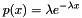#Boost C++ Libraries

...one of the most highly regarded and expertly designed C++ library projects in the world.

## Class template exponential_distribution

boost::random::exponential_distribution

## Synopsis

```// In header: <boost/random/exponential_distribution.hpp>

template<typename RealType = double>
class exponential_distribution {
public:
// types
typedef RealType input_type;
typedef RealType result_type;

// member classes/structs/unions

class param_type {
public:
// types
typedef exponential_distribution distribution_type;

// construct/copy/destruct
param_type(RealType = 1.0);

// public member functions
RealType lambda() const;

// friend functions
template<typename CharT, typename Traits>
friend std::basic_ostream< CharT, Traits > &
operator<<(std::basic_ostream< CharT, Traits > &, const param_type &);
template<typename CharT, typename Traits>
friend std::basic_istream< CharT, Traits > &
operator>>(std::basic_istream< CharT, Traits > &, const param_type &);
friend bool operator==(const param_type &, const param_type &);
friend bool operator!=(const param_type &, const param_type &);
};

// construct/copy/destruct
explicit exponential_distribution(RealType = 1.0);
explicit exponential_distribution(const param_type &);

// public member functions
RealType lambda() const;
RealType min() const;
RealType max() const;
param_type param() const;
void param(const param_type &);
void reset();
template<typename Engine> result_type operator()(Engine &) const;
template<typename Engine>
result_type operator()(Engine &, const param_type &) const;

// friend functions
template<typename CharT, typename Traits>
friend std::basic_ostream< CharT, Traits > &
operator<<(std::basic_ostream< CharT, Traits > &,
const exponential_distribution &);
template<typename CharT, typename Traits>
friend std::basic_istream< CharT, Traits > &
operator>>(std::basic_istream< CharT, Traits > &,
const exponential_distribution &);
friend bool operator==(const exponential_distribution &,
const exponential_distribution &);
friend bool operator!=(const exponential_distribution &,
const exponential_distribution &);
};```

## Description

The exponential distribution is a model of random distribution with a single parameter lambda.

It has### `exponential_distribution` public construct/copy/destruct

1. `explicit exponential_distribution(RealType lambda = 1.0);`

Constructs an `exponential_distribution` with a given lambda.

Requires: lambda > 0

2. `explicit exponential_distribution(const param_type & param);`

Constructs an `exponential_distribution` from its parameters

### `exponential_distribution` public member functions

1. `RealType lambda() const;`

Returns the lambda parameter of the distribution.

2. `RealType min() const;`

Returns the smallest value that the distribution can produce.

3. `RealType max() const;`

Returns the largest value that the distribution can produce.

4. `param_type param() const;`

Returns the parameters of the distribution.

5. `void param(const param_type & param);`

Sets the parameters of the distribution.

6. `void reset();`

Effects: Subsequent uses of the distribution do not depend on values produced by any engine prior to invoking reset.

7. `template<typename Engine> result_type operator()(Engine & eng) const;`

Returns a random variate distributed according to the exponential distribution.

8. ```template<typename Engine>
result_type operator()(Engine & eng, const param_type & param) const;```

Returns a random variate distributed according to the exponential distribution with parameters specified by param.

### `exponential_distribution` friend functions

1. ```template<typename CharT, typename Traits>
friend std::basic_ostream< CharT, Traits > &
operator<<(std::basic_ostream< CharT, Traits > & os,
const exponential_distribution & ed);```

Writes the distribution to a std::ostream.

2. ```template<typename CharT, typename Traits>
friend std::basic_istream< CharT, Traits > &
operator>>(std::basic_istream< CharT, Traits > & is,
const exponential_distribution & ed);```

Reads the distribution from a std::istream.

3. ```friend bool operator==(const exponential_distribution & lhs,
const exponential_distribution & rhs);```

Returns true iff the two distributions will produce identical sequences of values given equal generators.

4. ```friend bool operator!=(const exponential_distribution & lhs,
const exponential_distribution & rhs);```

Returns true iff the two distributions will produce different sequences of values given equal generators.